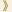HomeShaw Laureates2008Mathematical SciencesAutobiographyVladimir ArnoldI was born on 12 June 1937 in Odessa and studied at the Moscow University from 1954 to 1959.

I was a Candidate of physical-mathematical sciences, for the Thesis, resolving the Hilbert's 13-th problem, Applied Mathematics (Keldysh) Institute in 1961 and attained the physical-mathematical sciences doctor in 1963, for the Thesis on the stability of the Hamiltonian systems, at the same Institute. The graduated studies were supervised by A.N. Kolmogorov.

Since 1965 I have worked as a professor at the chair of differential equations of the mathematical-mechanical faculty of the Moscow State University and since 1986 also at the Steklov Mathematical Institute, Moscow. I was elected a member of the Russian Academy of Sciences in 1990.

I served as the vice-president of the International Union of Mathematicians (1999-2003), being also the President of the Moscow Mathematical Society.

The list of scientifical journals, on whose Editorial Boards I participated, includes, for instance:

Doklady RAN, Izvestia RAN, Russian Mathematical Surveys, Functional Analysis and its Applications, Functional Analysis and Other Mathematics, Proceedings of Petrovski Seminar, Inventiones Mathematicae, Physica D-Nonlinear Phenomena, Quantum, Bulletin des Sciences Mathematiques, Selecta, Journal of Geometry and Physics, Topological Methods in Nonlinear Analysis.

Being Moscow University's professor for 30 years, I worked also as the professor at the University Paris-Dauphine from 1993 to 2005 (remaining now its honorary professor).

I have published several dozens of books. Examples are:

Ergodic Problems of Classical Mechanics (with A. Avez);

• Ordinary Differential Equations;
• Mathematical Methods of Classical Mechanics;
• Geometrical Methods of theory of Ordinary Differential Equations;
• Catastrophes Theory;
• Singularities of Caustics and of Wave Fronts;
• Problems for Children from 5 to 15 years old;
• Huygens and Barrow, Newton and Hooke – first steps of calculus and of catastrophes theory;
• Yesterday and Long Ago;
• Contact Geometry and Wave Propagation;
• Lectures of Partial Derivatives equations;
• Pseudoperiodic Topology (with M. Kontsevitch and A. Zoritch);
• Mild and Soft Mathematical Models;
• Continued Fractions;
• Euler Groups and Geometric Progressions Arithmetics;
• Dynamics, Statistics and Projective Geometry of Galois Fields;
• New Obscurantism and Russia's Educational System;
• Is Mathematics Needed at Highschools;
• Geometry of Complex Numbers, Quaternions and Spins;
• Experimental Mathematics;
• What is Mathematics;
• Experimental Discoveries of Mathematical Facts;
• Science of Mathematics and Arts of Mathematicians;
• Geometry.

The above list contains 10 university textbooks.

Most known mathematical papers of mine deal with Hamiltonian systems (including the discovery of the “Arnold diffusion” and the creation of the symplectic topology).

My articles on the “quantum catastrophies theory” include the studies of the bifurcations of the caustics, based on my discovery of unexpected interrelations between the simple critical points of functions and simple Lie algebras (and also to Coxeter reflections' groups).

The real algebraic geometry of plane curves was related by me to the four-dimensional topology (and to quantum fields theory) – this discovery generated many studies by many mathematicians of the algebraic geometry part of the 16th problem of Hilbert.

My recent works on arithmetical turbulence provide unexpected statistical properties of the Young diagrams of the cycles of random permutations of N→∞ points.

Many domains of modern mathematics, generated by my articles, include, for instance:

Lagrange and Legendre cobordism theories (in symplectic and contact topologies);

• Statistics of the most frequent representations of finite groups;
• Ergodic theory of the segments' permutations;
• Planetary dynamo theory (in magnetohydrodynamics);
• Statistics of the higherdimensional continued fractions;
• Theory of singularities of the distribution of galaxies;
• Arnold's discovery of the “strange duality” of Lobachevsky triangles (leading to the mirror    symmetry theory of the quantum fields physics);
• Asymptotical statistics of the Fermat-Euler geometrical progressions of residues;
• Theory of the weak asymptotics (for the distributions of the solutions of Diophantine problems);
• Description of the boundary singularities of the optimal control problems (in terms of the
geometryof icosahedron);
• Topological Galois theory (of radical insolvability for the algebraic equations of degrees ≥ 5);
• Creation of the characteristic classes theories for the Braids and for the algebraic functions;
• Arnold's discovery of the topological reasons of the divergences of the permutation theory's series    (including the classification of the neigbourhoods and in the orbits spaces of dynamical systems);
• Asymptotical study of irreducible representations frequencies (in the eigenspaces of the Laplacian    on a symmetrical Riemannian manifold);
• Topological classification of the immersed smooth plane curves;
• Ergodic theory and projective geometry of Galois fields;
• Statistics of the convex polygons, whose vertices are integer points on the plane;
• Topological interpretation of the Maxwell's multipole formula for the spherical harmonics;
• Palindromicity theory for the periodic continued fractions of the quadric irrationalities    (x2+px+q=0);
• Arnold's discovery of the validity of the Gauss-Kuz'min statistics for the random periodic    continued fractions;
• Arnold's discovery of the violation of the Gauss-Kuz'min statistics for the periodic continued    fractions of eigenvalues of the random matrices (in SL(2, Z));
• Arnold's invention of the characteristic class, involved in the quantization conditions;
• Arnold's symplectic geometry theory of the Lagrange tore in completely integrable Hamilton    systems;
• The ergodic and number-theoretical “Arnold's cats” of physicists (F. Dyson, I. Persival, …).

To understand the natural interrelations between such different subjects as mentioned above, I recommend reading my articles (approximately 700) explaining these interrelations.

9 September 2008, Hong Kong